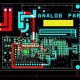# What Is Field Effect Transistor (FET)?

In this tutorial, We are going to learn about What Is Field Effect Transistor (FET)?

FET – Transistor or FET is a three terminal device, which use electric field to control the flow Of current through the device. And it is very useful in much application such as computer, automation and consumer electronics.

Working of Field Effect Transistor (FET)

This  FETs. So the three Terminals of the FET are knows as gate drain and source. So in FET, In this circuit can see the current used to flow between the drain and the source terminal. And this current can be controlled by voltage Generates the electric field within the device and by controlling this electric field or in way by Controlling this voltage we can control the flow of current through the device so basically in This field effect transistor, by controlling the electric field we can control the flow of current. And that is why it is known as the field effect transistor.

Difference between BJT & FET:

• The field effect transistor is the voltage control device that mains the input voltage between the you look at the BJT at the bipolar junction transistor, it is a current controlled device, where the input base current controls the output collector current so this is the one of the difference but in the field effect transistor and the bipolar junction transistor.
• The second difference is the FET is a unipolar device, while the BJT is bipolar device. Meaning that the BJT relies on the two types and the Charge the free electron and the holes. But the operation of the FET relies on either holes are electron. The FETs are smaller in size compared of the BJTs. And that’s why they are commonly used in the integrated circuits and  in terms of the power consumption the power consumption of the FET is less than BJT. And that’s why they are preferred in much high power application as well as in the computing.
• The main difference between the field Effect transistor and the BJT is that the input impedance of the field effect transistor is very high and due to that which is used as a buffer amplifier in many applications.

Application of Field Effect Transistor (FET)

• Amplifier
• Analog Switch
• Integrated Circuits
• Buffer Amplifier
• Oscillators

Application, the FETs Are used in almost all the application ,here the BJTs are used as A amplifier or oscillator in many application. application, particularly where the required power consumption should be minimum. So these are the few difference between the FET and the BJT.

Types of FET:

• JFET  –  The junction field transistor
• IG-FET – It is full form is Insulated Gate Field Effect Transistor